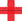top of page

## Video ResourcesIn this video, Luke or Lindsey takes students through a full Stats Medic EFFL lesson.

# Continuous Random Variables

##### Learning Targets
• Calculate and interpret the standard deviation of a discrete random variable.

• Use the probability distribution of a continuous random variable (uniform or Normal) to calculate the probability of an event.

bottom of page